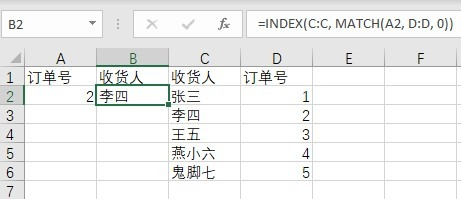# Excel的VLOOKUP公式计算提速方法

### 更新：真·一劳永逸的解决方法

Office 365在某个版本里从源头提高了VLOOKUP的执行效率，现在已经可以无脑VLOOKUP了，氪金竟然真的可以变强。

### 问题的症结

`=VLOOKUP(要查询的数据, 在哪几列查, 取第几列, FALSE)`

### 时间差不多能减半的处理方法

`=MATCH(要查询的数据, 在哪列查, 0)`

MATCH用来返回查找值在目标数组当中的位置，第三个参数0为精确匹配。也就是说，这个函数自己，可以直到你要找的值在匹配数据的第几行。

`=INDEX(从哪列取, 取第几行)`

`=INDEX(从哪列取, MATCH(要查询的数据, 在哪列查,0))`

“要查询的数据”是需要匹配的值，“在哪列查”是包含需要匹配的原始数据的那一列，“从哪列取”是需要取值的那一列。`=INDEX(C:C, MATCH(A2, D:D, 0))`

### 奇技淫巧

`=IF(VLOOKUP(要查询的数据, 在哪一列查（能升序排序）, TRUE)=要查询的数据, VLOOKUP(要查询的数据, 在哪几列查, 取第几列, TRUE), NA())``=IF(VLOOKUP(A2, C:C, TRUE)=A2, VLOOKUP(A2, C:D, 2, TRUE), NA())`